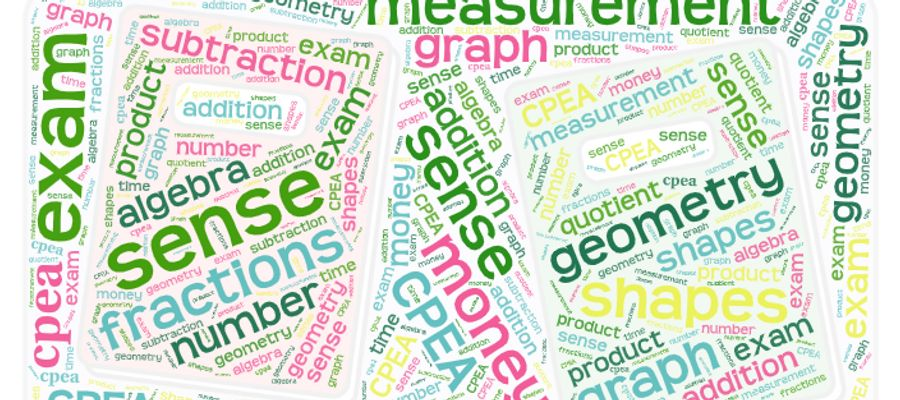# Mathematics 1

## CPEA Practice Math 1### Quiz Questions

• 1.
Study the image then answer the question
• A.
6 units
• B.
8 units
• C.
12 units
• 2.
What is the area of the parallelogram?
• A.
18m square
• B.
36 m square
• C.
13 m square
• 3.
Taylor pays \$5.25 for 0.5 yards of fabric. What is the cost per yard?
• A.
\$1.05
• B.
\$10.50
• C.
\$105.00
• 4.
Which list of numbers is ordered from least to greatest?
• A.
167213, 168455, 17400, 171633
• B.
160233, 171141, 175645, 176703
• C.
160233, 17645, 171141, 176703
• 5.
Which number is a multiple of 13?
• A.
70
• B.
43
• C.
39
• 6.
Which number is a prime number?
• A.
25
• B.
35
• C.
43
• 7.
Which step explains how to find the value of a in 6a = 72?
• A.
multiply both sides by 6
• B.
• C.
divide both sides by 6
• 8.
A tournament has 393 players with 3 players on each team. Which expression shows how to find the number of teams in the tournament?
• A.
393 × 3
• B.
393 + 3
• C.
393 ÷ 3
• 9.
When folded along the dotted lines, which geometric solid is formed?
• A.
cylinder
• B.
rectangular prism
• C.
triangular prism
• 10.
All of the sides of this triangle are equal. What kind of a triangle is this?
• A.
scalene
• B.
isoceles
• C.
equilateral

• 1.
Result 1